## conference papersJOURNAL OFAPPLIEDCRYSTALLOGRAPHY
ISSN: 1600-5767
Volume 40| Part s1| April 2007| Pages s18-s22

## Structure and dynamics of thin polymer films using synchrotron X-ray scattering

aDepartment of Physics, University of California San Diego, La Jolla, CA 92093, USA, bDepartment of Physics and Interdisciplinary Program of Integrated Biotechnology, Sogang University, Seoul 121-742, Republic of Korea, cDepartment of Physics, Northern Illinois University, DeKalb, IL 60115, USA, dDepartment of Materials Science and Engineering, SUNY at Stony Brook, Stony Brook, NY 11794, USA, eIntense Pulsed Neutron Source, Argonne National Laboratory, Argonne, IL 60439, USA, fAdvanced Photon Source, Argonne, IL 60439, USA, and gLANSCE, Los Alamos National Laboratory, Los Alamos, NM 87545, USA
*Correspondence e-mail: ssinha@physics.ucsd.edu

(Received 18 August 2006; accepted 16 February 2007; online 20 March 2007)

Recent measurements of the scattering function and of the dynamics of surface and interfacial fluctuations in thin supported molten films and bilayers using synchrotron X-ray diffuse scattering and photon correlation spectroscopy in reflection geometry are reported. The results for monolayer films thicker than four times of the radius of gyration of polystyrene show behavior of normal over-damped capillary waves expected for the surface fluctuations of a viscous liquid. However, thinner films show deviations indicating the need to account for viscoelasticity. The theory has been extended to the surface and interfacial modes in a bilayer film system. The results are discussed in terms of surface tension, viscosity and shear modulus. Also recent experiments to measure the isothermal compressibility of supported polystyrene films by studying bulk' scattering from the interior of the films is discussed.

### 1. Introduction

Thin polymer films are not only important in many technological applications but are also of great interest for basic science. The objective of our investigations was to use surface X-ray scattering from thin molten polymer films on substrates to examine how their properties might differ from those of bulk polymers. The dynamical properties of entangled chains in polymer melts have been the subject of much intense study over the last several years (de Gennes, 1979; de Gennes & Leger, 1982; Doi & Edwards, 1986; Milner & McLeish, 1998). Attempting to account for the manner in which the viscosity and the elastic moduli depend on quantities such as the molecular weight, entanglement length, frequency, etc. presents a considerable challenge for theory to explain in detail. The corresponding problem for polymer melts adsorbed on substrates in thin film form does not appear to have been addressed, although experimental data have been obtained using atomic force microscopy or the surface force apparatus. In this paper, we show that measurements of the wavevector dependence of the relaxation time for over-damped capillary waves on thin molten polystyrene (PS) films (thickness ≤ 2Rg, radius of gyration) provide a non-invasive method for measuring the surface tension, viscosity and shear modulus of these films and discuss the trends in the latter two quantities with molecular weight. In discussing the dynamics, we show that our current model also explains why earlier measurements of the static scattering function S(q) (q being the in-plane component of the wavevector transfer kfki of the scattering, where ki and kf are the incident and outgoing wavevectors of the X-ray photons, respectively) had to be interpreted in terms of brush-like models when the film thicknesses were comparable to Rg.

Certain physical properties of polymers such as the molecular mobility (Liu et al., 1997; Kajiyama et al., 1997) appear to differ from those of bulk polymers (de Gennes, 1979), in particular, when the thicknesses of the polymer films are comparable with the polymer radius of gyration (Rg). Thus Keddie et al. (1994) report a decrease of Tg for polymer films with thicknesses below ~2Rg. However, contrary results have been found to indicating no change or even an increase of Tg for thin polymer films (Wallace et al., 1995; Zheng et al., 1995; Frank et al., 1996; Zanten et al., 1996; Ge et al., 2000).

### 2. Surface fluctuations of monolayer films

In an earlier series of grazing-incidence diffuse-scattering X-ray measurements of supported polystyrene (PS) films quenched to room temperature from the molten state, Wang et al. (1999) reported that for film thicknesses comparable to Rg, the surface height–height correlation function was more characteristic of a film possessing a shear modulus than that of liquid capillary wave fluctuations. Thus these films exhibited a low in-plane q cutoff of the surface fluctuations which appeared to scale with the film thickness as h−3/4 instead of the h−2 behavior characteristic of a cutoff due to van der Waals interactions of a normal liquid film with the substrate. This behavior could be understood in terms of a model developed earlier for a polymer brush (Fredrickson et al., 1992). For much thicker PS films with h >> Rg, however, normal capillary wave behavior was observed in S(q).

We have now followed up those earlier measurements by carrying out dynamical measurements using X-ray photon correlation spectroscopy (XPCS) (Kim et al., 2003). This emerging technique applies the principles of dynamic light scattering in the X-ray regime. The short wavelength and slow time scales characteristic of XPCS provide us with a unique opportunity for measuring dynamics selectively from the surface fluctuations and extending the phase space accessible to scattering studies beyond what is accessible by light and neutron scattering.

Films were prepared by dissolving PS of several averaged molecular weights [Mw = 65, 123, 400, 650 and 900 kg mol−1 (Mw/Mn < 1.08)] in toluene and then spin-casting onto optically flat silicon substrates. The substrates were prepared to be hydrophobic. The thin-film samples were then annealed in vacuum for 24 h at 443 K to ensure complete solvent removal and afterwards quenched to room temperature. For each molecular weight, the thicknesses of the PS films were Rg, 2Rg and 4Rg. Rg of each molecular weight is shown in Table 1.

 Table 1The radius of gyration of PS for different molecular weights Rg = 0.67(N/6)1/2 nm, where N is the polymerization index
 Mw (kg mol−1) 65 123 400 650 900 Rg (nm) 6.8 9.4 17.0 21.7 25.5

XPCS experiments were performed at beamline 8-ID at the Advanced Photon Source (APS), Argonne National Laboratory, and employed monochromatic radiation with an X-ray energy of 7.5 keV. The schematic experimental set-up is shown in Fig. 1(a) and the schematic reflectivity geometry in XPCS is shown in Fig. 1(b). The off-specular diffuse scattering of the polymer surface was recorded with a direct-illumination charge-coupled device (CCD) camera located 3472 mm downstream of the sample. The beam dimensions were 20 × 20 µm2 and the speckle size at the detector was comparable to the CCD pixel size of 22.5 × 22.5 µm2. The polymer surface was thus partially coherently illuminated, giving rise to a speckled scattering pattern which varies in time as the surface modes experience random thermal fluctuations. The normalized intensity–intensity time autocorrelation function, g2 at a particular in-plane wavevector q, characterizing a particular surface fluctuation mode with wavelength 2π/q, is obtained from (Sutton, 2002; Grubel & Zontone, 2004)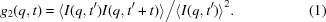For highly viscous liquid films, g2 = 1 + βexp[−2t/τ(q)] is used to fit the above autocorrelation function, where β is the speckle contrast and τ(q) is the relaxation time of the over-damped thermally induced capillary wave fluctuations of wavevector q.Figure 1 (a) Schematic set-up for surface X-ray photon correlation spectroscopy at the beamline 8-ID at the APS. Typical distances are: source–monochromator, 53 m; monochromator–sample, 2 m; sample–detector, 3.5 m. (b) The schematic reflectivity geometry in XPCS.

Our early set of XPCS studies (Kim et al., 2003) involved supported PS films of Mw 123 kg mol−1 at various thicknesses and temperatures between 423 K and 463 K, well above the glass transition temperature of 373 K. The behavior of the relaxation time τ as a function of q could be well explained by an expression derived by taking the small frequency limit of an expression for the imaginary part of the susceptibility for height fluctuations derived by Jackle for a liquid film of finite thickness and finite viscosity (Jackle, 1998). This expression is given by (Kim et al., 2003)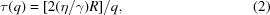where η is the viscosity of the liquid, γ is the surface tension, and the function R is given by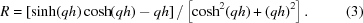From equation (2), one notices that there is a scaling relation, i.e. the quantity τ/h is only a function of the dimensionless quantity (qh) and the ratio of η/γ for all film thicknesses. This scaling relation was obeyed reasonably well (Kim et al., 2003) for all the thicknesses studied (which were all greater than 4Rg). Further, the obtained film viscosity appeared to be consistent with that obtained from bulk measurements. Thus, it appeared that the capillary wave dynamics of molten polymer films exhibited the normal behavior expected of a normal viscous liquid.

However, more recent XPCS measurements have been carried out on the dynamics of a series of thinner polymer films of various molecular weights with thicknesses ranging from 4Rg to Rg (Jiang et al., 2007). Fig. 2shows the function g2(q,t) obtained for PS films of Mw 123 kg mol−1 at 468 K and for different thicknesses of Rg, 2Rg, and 4Rg respectively and a given in-plane q. For h = Rg, no dynamics is observable, i.e. the chains are confined and stuck' on the surface.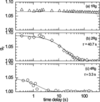Figure 2 The autocorrelation functions obtained from PS films (123 kg mol−1) with thickness of (a) 1Rg, (b) 2Rg and (c) 4Rg at 468 K for q = 7.7 × 10−3 nm−1. Solid lines are single exponential fits. The fitted relaxation time constants for the 2Rg and 4Rg films are labeled. There are no dynamics observed for the 1Rg film.

Fig. 3shows the derived relaxation times τ as a function of q for capillary waves on 2Rg and 4Rg films together with fits (dashed lines) using equation (2). While equation (2)fits the data for h = 4Rg, the results show deviations from equation (2)when the thickness is equal to 2Rg, regardless of molecular weight. This shows that it is not the absolute thickness but rather the ratio of thickness to Rg which affects the dynamics.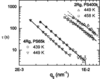Figure 3 Measured τ vs q for PS films with 65 kg mol−1, h = 4Rg, and 400 kg mol−1, h = 2Rg. Solid lines are fits with viscoelastic model [equation (4)] and dashed lines correspond to viscous model [equation (2)].

To explain the results for h = 2Rg, we calculated the surface dynamic susceptibility by generalizing the theory in Jackle (1998) for viscoelastic liquids. This can be done by replacing the viscosity by a complex frequency-dependent quantity η(ω) ≃ η + iμ/ω. Here μ is a shear modulus and η is the frequency. This yields a modified expression for τ(q),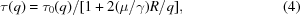where τ0(q) is the expression for τ(q) given by equation (2), and R is the function given by equation (3). This expression fits the results for thicknesses of 2Rg much better as shown in Fig. 3. In equation (4), when τ0(q) << η/μ, τ(q) becomes equal to τ0(q). This result is usually valid for large γ, thick films and small μ. On the other hand, when τ0(q) >> η/μ, τ(q) ~ η/μ. In general, the damping of capillary waves on the surfaces of thin polymer films exhibits intermediate properties between Newtonian liquids and Hookean solids. We define τmη/μ, the relaxation time that characterizes the time scale over which the viscoelastic liquid relaxes the stress undertaken. When polymer films are thin enough, the surface wave relaxation becomes wavevector independent and long wavelength capillary waves are completely suppressed. A corresponding calculation of the static susceptibility obtained from the dynamical theory above yields an expression for S(q) which is identical to that given by Fredrickson et al. (1992), although the expression they give for μ is that for a polymer brush which is not necessarily valid for an adsorbed polymer chain. Thus we can understand why for films with h of the order of 2Rg or less, the earlier measurements of S(q) for adsorbed polymer films (Wang et al., 1999; Seo et al., 2005) could be better explained by the model in Fredrickson et al. (1992) than by standard capillary wave theory.

The viscosities obtained for the films by fitting to the XPCS data show scaling with molecular weight with an exponent somewhat less than the 3.4 predicted by the reptation theory for entangled polymer melts, probably indicating a lower degree of entanglement as the film becomes thinner. The shear moduli obtained by fitting to the measured τ(q) for the above films were roughly consistent with bulk shear moduli for the same molecular weights. Thus it appeared that the normal models for viscoelastic films could account for the observed dynamics of thin PS films. However, there are indications that these models are not obeyed as the temperature begins to approach the glass transition temperature. Here measurements of the dynamics for PS films with large Mw values (up to ∼900 kg mol−1) and also very recent measurements for lower Mw values but at larger q values indicate that the effective film viscosity may be considerably lower than the corresponding bulk values. This may be consistent with observed reductions in Tg for thin supported polymer films. Further exploration of this regime is in process. These results, incidentally, point to the need for a more intense source of coherent X-rays in order to study dynamics at larger values of q. Such measurements are currently severely intensity limited. However, radiation-induced damage is a major concern and care has to be taken to avoid radiating the sample in one spot for a longer time than it takes to produce damage. This can often be managed by a fast shutter which can be periodically opened and closed if the time scale being measured is longer than the damage time.

### 3. Surface and interface fluctuations of bilayer films

The dynamics of the interface between two polymer films in a bilayer is also of interest for applications involving polymer blends, adhesives, etc. One can study this by using the grazing angle of incidence αi of the photon beam to probe both the free surface and the interface selectively. Thus for the free surface, if αi is less than the critical angle for total reflection, only the top surface is probed (provided the depth of the buried interface is much larger than the extinction depth), while if αi is chosen so that there is a node of the standing wave made by the incident wave and the reflected wave, while the interface is at, or close to, an anti-node then the buried interface is selectively probed. This can be shown using the distorted-wave Born approximation (DWBA) (Sinha et al., 1988) for the scattering.

We have carried out experiments (Hu et al., 2006) on a film of PS of Mw 200 kg mol−1 and thickness 100 nm on a film of poly(4-bromostyrene) (PBrS) of Mw 350 kg mol−1 and thickness 200 nm deposited on a silicon substrate. The bromination of the PBrS was 89%. The results (Fig. 4) show that there are two characteristic relaxation times associated with the free surface: a fast relaxation which is actually faster than the relaxation time for a single PS film of comparable thickness on Si and a slower mode with a q-independent relaxation time. The buried interface between the two films had fluctuations which essentially coincided in their τ vs q relation with the slow mode for the free PS surface.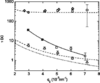Figure 4 Measured relaxation times at 468 K from bilayer (100 nm PS/200 nm PBrS): (open triangles) τ1, (open circles) τ2, (open diamonds) τ. Corresponding homolayers: (open squares) 100 nm PS. Theoretical models: single layer fit (solid line), low viscosity interface model (dashed line), slip model for top PS layer (dash–dot–dot line).

The dynamics of the bilayer was modelled as follows. The viscosity of the PS layer is assumed to be uniform throughout the film, and the PBrS layer is assumed immobile at the time scales of the experiment. A thin mixed layer is placed between the PS and PBrS films to account for the interface. Its viscosity is approximated as uniform. A value of 2.6 × 10−3 J m−2 was used for the interface tension between the mixed layer and the PS film. This was obtained from the magnitude of the surface roughness due to thermally excited, long wavelength capillary modes as measured by static diffuse X-ray scattering (Hu et al., 2005). Static scattering also provides the value of 6.6 nm for the thickness of the mixed layer (assuming the width is 2.35σ, with σ being the Gaussian roughness measured by neutron scattering). To discuss the dynamics of the coupled layers, we generalized the theory for the dynamics of the surface of a single viscoelastic film to the case of a bilayer film (Jiang et al., 2006). The linearized Navier–Stokes equation was used to solve for the fluid flow throughout two coupled layers. A non-slip boundary condition was assumed at the boundary between the mixed layer and the PBrS. At the PS/vacuum interface the surface tension balances the viscous stress. At the interface between the PS and the mixed layer the velocity is continuous and the difference in viscous stress between the two fluids is balanced by the interface tension. The viscoelastic properties of the polymer were taken into account by introducing a frequency dependent viscosity whose imaginary part involves the shear modulus. For the thick polystyrene film, the effect of the shear modulus is negligible and can be ignored. For the thin mixed layer, the addition of a shear modulus significantly changes the dynamics, as discussed above. This yields coupled modes for each surface, i.e., the free surface and the buried interface. The magnitude of the viscosity and shear modulus of the mixed layer in the resulting equation was then varied using nonlinear least-squares regression to obtain a best fit to the measured experimental relaxation times. This model provides a good fit, shown as the dashed line in Fig. 4, yielding μ ≃ 18 N m−2 and η ≃ 327 Ns m−2 for the mixed layer. The best-fit viscosity is only around 2% that of the PS layer. Thus, if one only considers the dynamics of the PS fast mode, the mixed layer can be viewed as introducing a finite slip length to the PS/PBrS interface. The dynamics of the slow mode of the top surface, which is nearly identical to the dynamics of the mixed layer, shows almost no dependence on wavevector. This wavevector dependence cannot be explained by a thin viscous layer, since by equation (2), a q−4 dependence for τ is predicted for a thin film. A simple scaling argument also predicts the same dependence for a thin film (de Gennes et al., 2002). However, the inclusion of the shear modulus term produces a flattening of the spectrum. The value of μ obtained from the fits was of the same magnitude of the shear modulus for bulk PS at comparable time scales (Graessley, 2004) and also of the same magnitude as the values needed to fit the relaxation times of the thin single-layer polymer films at comparable temperatures discussed in the previous section.

While the model described above does give a good fit to the data, it is clear that it can only be an approximation to the actual interfacial region, since the mixed layer is unlikely to have a uniform viscosity. Furthermore, the approximation of a surface tension is not precisely applicable over length scales comparable with the thickness of the interface. Nevertheless, the present results should provide a significant improvement over previous rheological results. Here we not only can imply the existence of slip between PS and PBrS, but we have estimates for the thickness, viscosity, surface tension and shear modulus of the mixed region. It seems reasonable to speculate that the origin of the low viscosity in the mixed region is similar to the causes of the reduced glass transition at the free surface of PS first observed by Keddie et al. (1994), since one might expect that the viscosity above Tg should decrease if Tg decreases. It would be interesting to investigate if thin PS films on top of PBrS show a reduced glass transition temperature closer to a freely suspended film, than a supported one. Finally, we note that experiments on the mobility of gold nanoparticles at the polymer–polymer interface in a bilayer system of PtBA/PtBA (Narayanan et al., 2005) have also shown anomalously high mobility. This may be similarly related to a region of reduced viscosity as we have found here.

### 4. Interior fluctuations of polymer films

At grazing angles of incidence, the scattering can be treated as coming from the surface. However, if the angle the scattered beam makes with the surface is made large, bulk' fluctuations from the interior of the polymer film are also significant and can be studied. In fact, in the DWBA (Sinha et al., 1988), the scattering may be written as the sum of two parts: a surface' part given by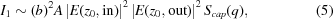where E(z, in) and E(z, out) are the incident electric field of the incident and outgoing radiation, respectively, at height z in the film, and a bulk' part given by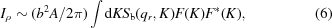where Sb(q) is the bulk structure factor and F(K) is the Fourier transform of [E(z, in)E*(z, out)]. Sb(q) can be approximated at small values of q by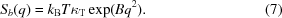The sum of these two terms in equations (5)and (6)was fitted to the scattering from a molten PS film (Mw 129 kg mol−1) as a function of grazing angle of incidence and of temperatures for a large scattering angle at an in-plane q vector of 1.34 nm−1. An example is shown in Fig. 5. It may be seen that while the surface term predominates at small grazing angles of incidence, the bulk' scattering from the film becomes important at large grazing angles of incidence.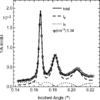Figure 5 Diffuse scattering cross-sections of a 100 nm PS film on Si at 373 K as a function of grazing angle of incidence. The solid line is the fit to the model discussed in the text. The contributions from the surface (dotted line) and bulk (dashed line) terms are shown separately.

The corresponding obtained values of Sb(0) are shown in Fig. 6. It can be seen that a break in the temperature dependence corresponding to a glass transition temperature can be observed in the thickest film but washes out as the film becomes thinner. It may also be seen that the isothermal compressibility κT (related to Sb(0) by equation (7)) increases as the films become thinner. This is presumably due to lower entanglement densities in the thinner films.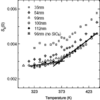Figure 6 Sb(0) vs temperature for PS films of different thicknesses. Sb(0) is extrapolated using equation (7). The intercept of the solid lines indicates the Tg (369 ± 8 K) for a 122 nm film.

### Acknowledgements

This work was supported by the US Department of Energy, BES Programs, NSF Grant No. DMR-0209542, the Ministry of Science and Technology of Korea (the International Cooperation Research Program) and 2003 Special Research Fund from Sogang University. The Advanced Photon Source is supported by the US Department of Energy, Office of Basic Science, under Contract No. DE-AC02-06CH11357.

### ReferencesJOURNAL OFAPPLIEDCRYSTALLOGRAPHY